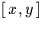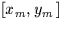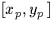Next: SLA_RANDOM - Random Number
Up: SUBPROGRAM SPECIFICATIONS
Previous: SLA_PVOBS - Observatory Position & Velocity

## SLA_PXY - Apply Linear Model

ACTION:
Given arrays of expected and measuredcoordinates, and a linear model relating them (as produced by sla_FITXY), compute the array of predicted coordinates and the RMS residuals.

CALL:
CALL sla_PXY (NP,XYE,XYM,COEFFS,XYP,XRMS,YRMS,RRMS)

GIVEN:

 I NP number of samples XYE D(2,NP) expectedfor each sample XYM D(2,NP) measuredfor each sample COEFFS D(6) coefficients of model (see below)

RETURNED:

 D(2,NP) XYP predictedfor each sample XRMS D RMS in X YRMS D RMS in Y RRMS D total RMS (vector sum of XRMS and YRMS)

NOTES:
1.
The model is supplied in the array COEFFS. Naming the six elements of COEFFS a,b,c,d,e & f, the model transforms measured coordinatesinto predicted coordinatesas follows:
xp = a + bxm + cym
yp = d + exm + fym
2.
The residuals are (xp-xe) and (yp-ye).
3.
If NP is less than or equal to zero, no coordinates are transformed, and the RMS residuals are all zero.
4.